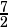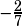Can you work out problem #11 of section four in Official SAT #5?

Sure. The math concept you need to know here is that perpendicular lines have negative reciprocal slopes. For example, if one line has a slope of, then a line perpendicular to it must have a slope of.

In this case, none of the lines are given to you in slope-intercept form, so your first step should be to put at least the first line you’re given into that form.That tells you that the slope of the given line is, so the slope you need to find in the answer choices is.

They do you a real favor in the answer choices by only using 3 and 2 as coefficients together in one choice. So just from the get-go you should be leaning choice A. Let’s just put it in slope-intercept form to be sure.Yep, that’s what we wanted. We’re all done!